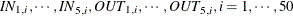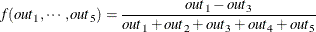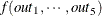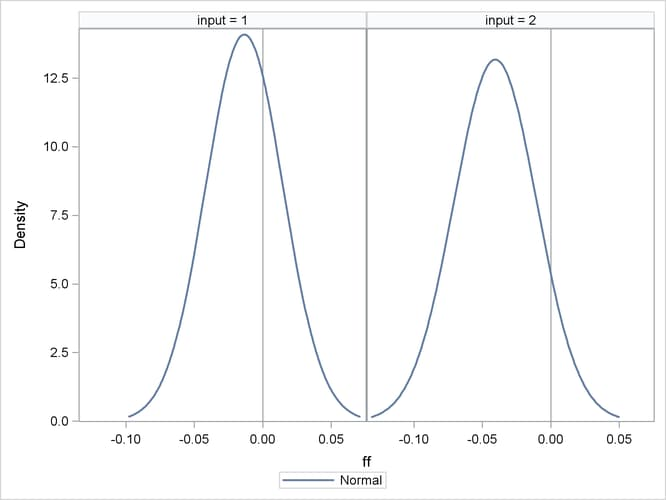## Getting Started: SIMNORMAL Procedure

The following example illustrates the use of PROC SIMNORMAL to generate variable values conditioned on a set of related or correlated variables.

Suppose you are given a sample of size 50 from ten normally distributed, correlated random variables,. The first five variables represent input variables for a chemical manufacturing process, and the last five are output variables.

First, the data are input and the correlation structure is determined by using PROC CORR, as in the following statements. The results are shown in Figure 86.1.

data a ;
input in1-in5 out1-out5 ;
datalines ;
9.3500    10.0964     7.3177    10.3617    10.3444    9.4612
10.7443     9.9026     9.0144    11.7968
7.8599    10.4560    10.0075     8.5875    10.0014   10.3869

... more lines ...

8.9174     9.9623     9.5742     9.9713
run ;

proc corr data=a cov nocorr outp=outcov ;
var in1-in5 out1-out5 ;
run ;


Figure 86.1: Correlation of Chemical Process Variables

The CORR Procedure

10 Variables: in1 in2 in3 in4 in5 out1 out2 out3 out4 out5

Covariance Matrix, DF = 49
in1 in2 in3 in4 in5 out1 out2 out3 out4 out5
in1 1.019198331 0.128086799 0.291646382 0.327014916 0.417546732 0.097650713 0.206698403 0.516271121 0.118726106 0.261770905
in2 0.128086799 1.056460818 0.143581799 0.095937707 0.104117743 0.056612934 -0.121700731 0.266581451 0.092288067 -0.020971411
in3 0.291646382 0.143581799 1.384051249 0.058853960 0.326107730 0.093498839 0.078294087 0.481576554 0.057816322 0.259053423
in4 0.327014916 0.095937707 0.058853960 1.023128678 0.347916864 0.022915645 0.125961491 0.179627237 0.075028230 0.078147576
in5 0.417546732 0.104117743 0.326107730 0.347916864 1.606858140 0.360270318 0.297046593 0.749212945 0.220196337 0.349618466
out1 0.097650713 0.056612934 0.093498839 0.022915645 0.360270318 0.807007554 0.217285879 0.064816340 -0.053931448 0.037758721
out2 0.206698403 -0.121700731 0.078294087 0.125961491 0.297046593 0.217285879 0.929455806 0.206825664 0.138551008 0.054039499
out3 0.516271121 0.266581451 0.481576554 0.179627237 0.749212945 0.064816340 0.206825664 1.837505268 0.292963975 0.165910481
out4 0.118726106 0.092288067 0.057816322 0.075028230 0.220196337 -0.053931448 0.138551008 0.292963975 0.832831377 -0.067396486
out5 0.261770905 -0.020971411 0.259053423 0.078147576 0.349618466 0.037758721 0.054039499 0.165910481 -0.067396486 0.697717191

Simple Statistics
Variable N Mean Std Dev Sum Minimum Maximum
in1 50 10.18988 1.00955 509.49400 7.63500 12.58860
in2 50 10.10673 1.02784 505.33640 8.12580 13.78310
in3 50 10.14888 1.17646 507.44420 7.31770 12.40080
in4 50 10.03884 1.01150 501.94200 7.40490 11.99060
in5 50 10.22587 1.26762 511.29340 7.23350 12.93360
out1 50 9.85347 0.89834 492.67340 8.01220 12.24660
out2 50 9.96857 0.96408 498.42840 7.76420 12.09450
out3 50 10.29588 1.35555 514.79410 7.29660 13.74200
out4 50 10.15856 0.91260 507.92780 8.43090 12.45230
out5 50 10.26023 0.83529 513.01130 7.86060 11.96000

After the mean and correlation structure are determined, any subset of these variables can be simulated. Suppose you are interested in a particular function of the output variables for two sets of values of the input variables for the process. In particular, you are interested in the mean and variability of the following function over 500 runs of the process conditioned on each set of input values:Although the distribution of these quantities could be determined theoretically, it is simpler to perform a conditional simulation by using PROC SIMNORMAL.

To do this, you first append a _TYPE_=’COND’ observation to the covariance data set produced by PROC CORR for each group of input values:

data cond1 ;
_TYPE_='COND' ;
in1 = 8    ;
in2 = 10.5 ;
in3 = 12   ;
in4 = 13.5 ;
in5 = 14.4 ;
output ;
run ;

data cond2 ;
_TYPE_='COND' ;
in1 = 15.4 ;
in2 = 13.7 ;
in3 = 11 ;
in4 = 7.9 ;
in5 = 5.5 ;
output ;
run ;


Next, each of these conditioning observations is appended to a copy of the OUTP=OUTCOV data from the CORR procedure, as in the following statements. A new variable, INPUT, is added to distinguish the sets of input values. This variable is used as a BY variable in subsequent steps.

data outcov1 ;
input=1 ;
set outcov cond1 ;
run ;

data outcov2 ;
input=2 ;
set outcov cond2 ;
run ;


Finally, these two data sets are concatenated:

data outcov ;
set outcov1 outcov2 ;
run ;
proc print data=outcov ;
where (_type_ ne 'COV') ;
run ;


Figure 86.2 shows the added observations.

Figure 86.2: OUTP= Data Set from PROC CORR with _TYPE_=COND Observations Appended

Obs input _TYPE_ _NAME_ in1 in2 in3 in4 in5 out1 out2 out3 out4 out5
11 1 MEAN   10.1899 10.1067 10.1489 10.0388 10.2259 9.8535 9.9686 10.2959 10.1586 10.2602
12 1 STD   1.0096 1.0278 1.1765 1.0115 1.2676 0.8983 0.9641 1.3555 0.9126 0.8353
13 1 N   50.0000 50.0000 50.0000 50.0000 50.0000 50.0000 50.0000 50.0000 50.0000 50.0000
14 1 COND   8.0000 10.5000 12.0000 13.5000 14.4000 . . . . .
25 2 MEAN   10.1899 10.1067 10.1489 10.0388 10.2259 9.8535 9.9686 10.2959 10.1586 10.2602
26 2 STD   1.0096 1.0278 1.1765 1.0115 1.2676 0.8983 0.9641 1.3555 0.9126 0.8353
27 2 N   50.0000 50.0000 50.0000 50.0000 50.0000 50.0000 50.0000 50.0000 50.0000 50.0000
28 2 COND   15.4000 13.7000 11.0000 7.9000 5.5000 . . . . .

You now run PROC SIMNORMAL, specifying the input data set and the VAR and COND variables. Note that you must specify a TYPE=COV or TYPE=CORR for the input data set. PROC CORR automatically assigns a TYPE=COV or TYPE=CORR attribute for the OUTP= data set. However, since the intermediate DATA steps that appended the _TYPE_=’COND’ observations turned off this attribute, an explicit TYPE=CORR in the DATA= option in the PROC SIMNORMAL statement is needed.

The specification of PROC SIMNORMAL now follows from the problem description. The condition variables are IN1–IN5, the analysis variables are OUT1–OUT5, and 500 realizations are required. A seed value can be chosen arbitrarily, or the system clock can be used. Note that in the following statements, the simulation is done for each of the values of the BY variable INPUT:

proc simnormal data=outcov(type=cov)
out = osim
numreal = 500
seed = 33179
;
by input ;
var out1-out5 ;
cond in1-in5 ;
run;

data b;
set osim ;
denom = sum(of out1-out5) ;
if abs(denom) < 1e-8 then ff = . ;
else ff = (out1-out3)/denom ;
run ;


The DATA step that follows the simulation computes the function; in the following statements the UNIVARIATE procedure computes the simple statistics for this function for each set of conditioning input values. This is shown in Figure 86.3, and Figure 86.4 shows the distribution of the function values for each set of input values by using the SGPANEL procedure.

proc univariate data=b ;
by input ;
var ff ;
run ;
title ;
proc sgpanel data=b ;
panelby input ;
REFLINE 0 / axis= x ;
density ff ;
run ;


Figure 86.3: Simple Statistics for ff for Each Set of Input Values

The UNIVARIATE Procedure
Variable: ff

Moments
N 500 Sum Weights 500
Mean -0.0134833 Sum Observations -6.7416303
Std Deviation 0.02830426 Variance 0.00080113
Skewness 0.56773239 Kurtosis 1.31522925
Uncorrected SS 0.49066351 Corrected SS 0.39976435
Coeff Variation -209.92145 Std Error Mean 0.0012658

Basic Statistical Measures
Location Variability
Mean -0.01348 Std Deviation 0.02830
Median -0.01565 Variance 0.0008011
Mode . Range 0.21127
Interquartile Range 0.03618

Tests for Location: Mu0=0
Test Statistic p Value
Student's t t -10.6519 Pr > |t| <.0001
Sign M -106 Pr >= |M| <.0001
Signed Rank S -33682 Pr >= |S| <.0001

Quantiles (Definition 5)
Quantile Estimate
100% Max 0.11268600
99% 0.07245656
95% 0.03270269
90% 0.02064338
75% Q3 0.00370322
50% Median -0.01564850
25% Q1 -0.03247389
10% -0.04716239
5% -0.05572806
1% -0.07201126
0% Min -0.09858350

Extreme Observations
Lowest Highest
Value Obs Value Obs
-0.0985835 471 0.0750538 22
-0.0908179 472 0.0794747 245
-0.0802423 90 0.0840160 48
-0.0760645 249 0.1004812 222
-0.0756070 226 0.1126860 50

The UNIVARIATE Procedure
Variable: ff

Moments
N 500 Sum Weights 500
Mean -0.0405913 Sum Observations -20.295631
Std Deviation 0.03027008 Variance 0.00091628
Skewness 0.1033062 Kurtosis -0.1458848
Uncorrected SS 1.28104777 Corrected SS 0.4572225
Coeff Variation -74.57289 Std Error Mean 0.00135372

Basic Statistical Measures
Location Variability
Mean -0.04059 Std Deviation 0.03027
Median -0.04169 Variance 0.0009163
Mode . Range 0.18332
Interquartile Range 0.04339

Tests for Location: Mu0=0
Test Statistic p Value
Student's t t -29.985 Pr > |t| <.0001
Sign M -203 Pr >= |M| <.0001
Signed Rank S -58745 Pr >= |S| <.0001

Quantiles (Definition 5)
Quantile Estimate
100% Max 0.06101208
99% 0.02693796
95% 0.01008202
90% -0.00111776
75% Q3 -0.01847726
50% Median -0.04169199
25% Q1 -0.06187039
10% -0.07798499
5% -0.08606522
1% -0.11026564
0% Min -0.12231183

Extreme Observations
Lowest Highest
Value Obs Value Obs
-0.122312 937 0.0272906 688
-0.119884 980 0.0291769 652
-0.113512 920 0.0388217 670
-0.112345 523 0.0477261 845
-0.110497 897 0.0610121 632

Figure 86.4: Frequency Plot for ff for Each Set of Input Values